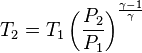## Homework Statement

1 kg of H20 goes through a reversible adiabatic proces from 220kPa, 200C to 50C in the final state. What is the pressure final and volume final?

Assume S1=s2

## Homework Equations

Conservation of Energy

## The Attempt at a Solution

Found S to be 7.5080, was thinking I could find this relating to 30°C, but missing something.

T_2 = T_1 \left( \frac{P_2}{P_1} \right)^{\frac{\gamma-1}{\gamma}}

Found that, but dont really know what the gammas represent?Use your steam tables or temperature-entropy diagram for water. Your initial condition is super heated. So you will need to look in the super heated steam tables. Find P1 and T1 in the tables to determine entropy (s1). You might need to do some interpolation to find S1 because the pressures in the tables may not be the exactly what you are looking for. Go to the saturated water tables for T2 and S1. You are saturated if S1 is between Sf and Sg for that temperature. It looks like you are. Read P2 from the table.

Wow, I was way over complicating that, thanks so much RTW69!!!

Then I took the specific volume X the mass of the water and got the volume (just in case someone looks at this in the future).